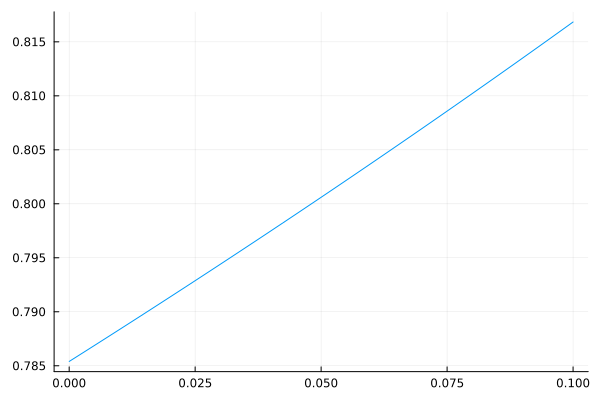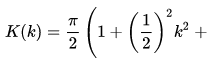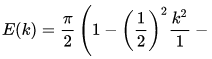# Problem with elliptic integrals

using Plots

using SpecialFunctions
# using Elliptic

K = ellipk # Computes Complete Elliptic Integral of 1st kind K(m)
E = ellipe # Computes Complete Elliptic Integral of 2nd kind E(m)

psi0(k) = (K(k) - E(k)) / k

@show psi0(1e-8) # psi0(1.0e-8) = 0.7853981909278218

x = range(1e-5, 0.1, length=1000)
y = psi0
plot(x, y; label = :none)The results are the same whether I use SpecialFunctions or Elliptic

Now, from Wikipedia,We neglect here the higher terms for k approaching zero (by cropping screenshot)

Thus, for small k
(K(k) - E(k)) / k ≈ k * π / 4  which tends to zero with k tending to zero.
Not to 0.785...From the docs the elliptic integrals are defined using m as argument, not k, with k^{2} = m. Which means the numerator in your function is approximately m\pi/4, denominator m, hence you get a number approaching \pi/4.

2 Likes